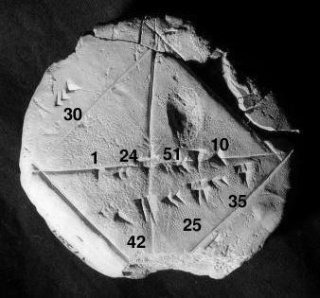# Numerical analysis

Topic | v1 | created by jjones |Description

Numerical analysis is the study of algorithms that use numerical approximation (as opposed to symbolic manipulations) for the problems of mathematical analysis (as distinguished from discrete mathematics). Numerical analysis naturally finds application in all fields of engineering and the physical sciences, but in the 21st century also the life sciences, social sciences, medicine, business and even the arts have adopted elements of scientific computations. The growth in computing power has revolutionized the use of realistic mathematical models in science and engineering, and subtle numerical analysis is required to implement these detailed models of the world.

##### Relations

Computer science is the study of computation and information. Computer science deals with theory of c...##### Resources